#### Dictionary of Special Options

The notes Traditional Graphics and Line Printer identify options that apply only to traditional graphics and line printers charts, respectively.

ALPHA=value

requests probability limits. If you specify ALPHA=, the control limits are computed so that the probability isthat a single EWMA exceeds its control limits. The value ofcan range between 0 and 1. This assumes that the process is in statistical control and that the data follow a normal distribution. For the equations used to compute probability limits, see Control Limits.

Note the following:

• As an alternative to specifying ALPHA=, you can readfrom the variable _ALPHA_ in a LIMITS= data set by specifying the READALPHA option.

• As an alternative to specifying ALPHA=(or reading _ALPHA_ from a LIMITS= data set), you can request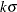control limits by specifying SIGMAS=k (or reading _SIGMAS_ from a LIMITS= data set).

If you specify neither the ALPHA= option nor the SIGMAS= option, the procedure computes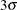control limits by default.

ASYMPTOTIC

requests constant upper and lower control limits based on the following asymptotic expressions: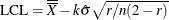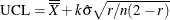Here r is the weight parameter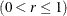, and n is the nominal sample size associated with the control limits. Substitute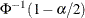for k if you specify probability limits with the ALPHA= option. When you do not specify the ASYMPTOTIC option, the control limits are computed using the exact formulas in Table 9.3. Use the ASYMPTOTIC option only if all the subgroup sample sizes are the same or if you specify LIMITN=n. See Example 9.2.

CMEANSYMBOL=color

Traditional Graphicsspecifies the color for the symbol requested with the MEANSYMBOL= option.

LIMITN=n
LIMITN=VARYING

specifies either a fixed or varying nominal sample size for the control limits.

If you specify LIMITN=n, EWMAs are calculated and displayed only for those subgroups with a sample size equal to n, unless you also specify the ALLN option, which causes all the EWMAs to be calculated and displayed. By default (or if you specify LIMITN=VARYING), EWMAs are calculated and displayed for all subgroups, regardless of sample size.

MEANCHAR=’character

Line Printerspecifies a character used to plot the subgroup mean for each subgroup. By default, subgroup means are not plotted.

MEANSYMBOL=keyword

Traditional Graphicsspecifies a symbol used to plot the subgroup mean for each subgroup. By default, subgroup means are not plotted.

MU0=value

specifies a known (standard) value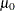for the process mean. By default,is estimated from the data. See Example 9.1.

Note: As an alternative to specifying MU0=, you can read a predetermined value forfrom the variable _MEAN_ in a LIMITS= data set.

specifies that control limit parameters for each process listed in the EWMACHART statement are not to be read from the LIMITS= data set specified in the PROC MACONTROL statement. The NOREADLIMITS option is available only in SAS 6.10 and later releases.

The following example illustrates the NOREADLIMITS option:

proc macontrol data=Pistons limits=Diamlim;
ewmachart Diameter*Hour;
run;


The first EWMACHART statement reads the control limits from the first observation in the data set Diamlim for which the variable _VAR_ is equal to 'Diameter' and the variable _SUBGRP_ is equal to 'Hour'. The second EWMACHART statement computes estimates of the process mean and standard deviation for the control limits from the measurements in the data set Pistons. Note that the second EWMACHART statement is equivalent to the following statements, which would be more commonly used:

proc macontrol data=Pistons;
ewmachart Diameter*Hour / weight=0.3;
run;


specifies that the variable _ALPHA_, rather than the variable _SIGMAS_, is to be read from a LIMITS= data set when both variables are available in the data set. Thus the limits displayed are probability limits. If you do not specify the READALPHA option, then _SIGMAS_ is read by default.

reads control limit parameters from a LIMITS= data set (specified in the PROC MACONTROL statement) for each process listed in the EWMACHART statement.

The control limit parameters for a particular process are read from the first observation in the LIMITS= data set for which

• the value of _VAR_ matches process

• the value of _SUBGRP_ matches the subgroup-variable

• the value of _INDEX_ matches value

The value can be up to 48 characters and must be enclosed in quotes.

specifies that control limit parameters are to be read from a LIMITS= data set specified in the PROC MACONTROL statement. The parameters for a particular process are read from the first observation in the LIMITS= data set for which

• the value of _VAR_ matches process

• the value of _SUBGRP_ matches the subgroup variable

The use of the READLIMITS option depends on which release of SAS/QC software you are using.

• In SAS 6.10 and later releases, the READLIMITS option is not necessary. To read control limits parameters as described previously, you simply specify a LIMITS= data set. However, even though the READLIMITS option is redundant, it continues to function as in earlier releases.

• In SAS 6.09 and earlier releases, you must specify the READLIMITS option to read control limits parameters as described previously. If you specify a LIMITS= data set without specifying the READLIMITS option (or the READINDEX= option), the control limits are computed from the data and the value of the weight parameter is specified with the WEIGHT= option.

RESET

requests that the value of the EWMA be reset after each out-of-control point. Specifically, when a point exceeds the control limits, the EWMA for the next subgroup is computed as the weighted average of the subgroup mean and the overall mean. By default, the EWMAs are not reset.

SIGMA0=value

specifies a known (standard) value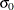for the process standard deviation. The value must be positive. By default, the MACONTROL procedure estimatesfrom the data using the formulas given in Methods for Estimating the Standard Deviation.

Note: As an alternative to specifying SIGMA0=, you can read a predetermined value forfrom the variable _STDDEV_ in a LIMITS= data set.

SIGMAS=value

specifies the width of the control limits in terms of the multiple k of the standard error of the plotted EWMAs on the chart. The value of k must be positive. By default, k = 3 and the control limits arelimits.

WEIGHT=value

specifies the weight r assigned to the most recent subgroup mean in the computation of the EWMA (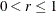). The WEIGHT= option is required unless you read control limit parameters from a LIMITS= data set or a TABLE= data set. See the section Choosing the Value of the Weight Parameter for details.